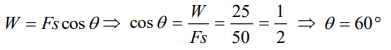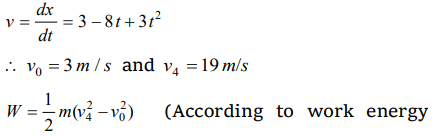## Work, Energy and Power Questions and Answers Part-1

1. A body of mass m is moving in a circle of radius r with a constant speed v. The force on the body is $\frac{mv^{2}}{r}$ and is directed towards the centre. What is the work done by this force in moving the body over half the circumference of the circle
a) $\frac{mv^{2}}{\pi r^{2}}$
b) Zero
c) $\frac{mv^{2}}{ r^{2}}$
d) $\frac{\pi r^{2}}{ mv^{2}}$

Explanation:2. If the unit of force and length each be increased by four times, then the unit of energy is increased by
a) 16 times
b) 8 times
c) 2 times
d) 4 times

Explanation: Work = Force × Displacement (length)
If unit of force and length be increased by four times then the unit of energy will increase by 16 times

3. A man pushes a wall and fails to displace it. He does
a) Negative work
b) Positive but not maximum work
c) No work at all
d) Maximum work

Explanation: No displacement is there

4. The same retarding force is applied to stop a train. The train stops after 80 m. If the speed is doubled, then the distance will be
a) The same
b) Doubled
c) Halved
d) Four times

Explanation:5. A body moves a distance of 10 m along a straight line under the action of a force of 5 N. If the work done is 25 joules, the angle which the force makes with the direction of motion of the body is
a) 0°
b) 30°
c) 60°
d) 90°

Explanation:6. You lift a heavy book from the floor of the room and keep it in the book-shelf having a height 2 m. In this process you take 5 seconds. The work done by you will depend upon
a) Mass of the book and time taken
b) Weight of the book and height of the bookshelf
c) Height of the book-shelf and time taken
d) Mass of the book, height of the book-shelf and time taken

Explanation: Work done = Force × displacement = Weight of the book × Height of the book shelf

7. A body of mass m kg is lifted by a man to a height of one metre in 30 sec. Another man lifts the same mass to the same height in 60 sec. The work done by them are in the ratio
a) $1:2$
b) $1:1$
c) $2:1$
d) $4:1$

Explanation: Work done does not depend on time

8. A force acts on a 30 gm particle in such a way that the position of the particle as a function of time is given by $x=3t-4t^{2}+t^{3}$ , where x is in metres and t is in seconds. The work done during the first 4 seconds is
a) 5.28 J
b) 450 mJ
c) 490 mJ
d) 530 mJ

Explanation:9. A body of mass 10 kg is dropped to the ground from a height of 10 metres. The work done by the gravitational force is $\left(g=9.8m\diagup s^{2}\right)$
a) – 490 Joules
b) + 490 Joules
c) – 980 Joules
d) + 980 Joules